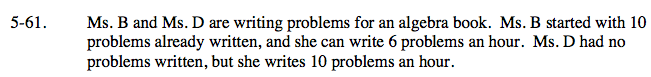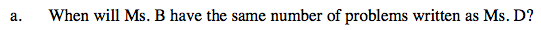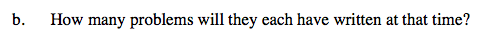### Home > CAAC > Chapter 5 > Lesson 5.1.6 > Problem5-61

5-61.Write down the problem as two equations in which x equals the time in hours and y equals the number of problems.

Ms. B: y = 6x + 10
Ms. D: y = 10x + 0

Use the Equal Values Method by setting the equations equal to each other and solving for x to find the time.

Substitute x into either of the original equations above and solve for y to find how many problems they will each have written at that time.